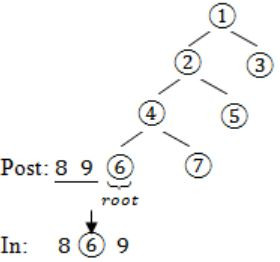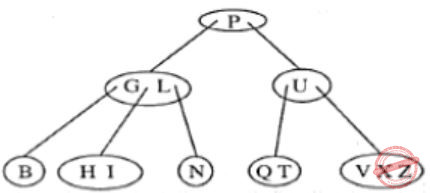## Trees

 Question 1

The postorder traversal of a binary tree is 8, 9, 6, 7, 4, 5, 2, 3, 1. The inorder traversal of the same tree is 8, 6, 9, 4, 7, 2, 5, 1, 3. The height of a tree is the length of the longest path from the root to any leaf. The height of the binary tree above is _______.

 A 1 B 2 C 3 D 4
Data-Structures       Trees       GATE 2018       Video-Explanation
Question 1 Explanation:
Post – 8 9 6 7 4 5 2 3 1
In – 8 6 9 4 7 2 5 1 3
Post: 8 9 6 7 4 5 2 3 1→(root)
In: 8 6 9 4 7 2 5→(left subtree) 13→(right subtree)Question 2

Let T be a tree with 10 vertices. The sum of the degrees of all the vertices in T is _________.

 A 18 B 19 C 20 D 21
Data-Structures       Trees       GATE 2017 [Set-1]       Video-Explanation
Question 2 Explanation:
Sum of degrees of all vertices is double the number of edges.
A tree, with 10 vertices, consists n - 1, i.e. 10 - 1 = 9 edges.
Sum of degrees of all vertices = 2(#edges)
= 2(9)
= 18
 Question 3

The number of ways in which the numbers 1, 2, 3, 4, 5, 6, 7 can be inserted in an empty binary search tree, such that the resulting tree has height 6, is _________.

Note: The height of a tree with a single node is 0.
 A 64 B 65 C 66 D 67
Data-Structures       Trees       GATE 2016 [Set-2]       Video-Explanation
Question 3 Explanation:
To get the tree of height 6, every level should contain only 1 node.
So to get such tree at each level we should have either maximum or minimum element from remaining numbers till that level. So no. of binary search tree possible is,
1st level - 2 (maximum or minimum)

2nd level - 2

3rd level - 2

4th level - 2

5th level - 2

6th level - 2

7th level - 2
= 2 × 2 × 2 × 2 × 2 × 2 × 1
= 26
= 64
 Question 4

The height of a tree is the length of the longest root-to-leaf path in it. The maximum and minimum number of nodes in a binary tree of height 5 are

 A 63 and 6, respectively B 64 and 5, respectively C 32 and 6, respectively D 31 and 5, respectively
Data-Structures       Trees       GATE 2015 [Set-1]
Question 4 Explanation:
Maximum number of nodes in a binary tree of height h is,
2h+1 - 1 = 25+1 - 1 = 63
Minimum number of nodes in a binary tree of height h is
h + 1 = 5 + 1 = 6
 Question 5

Consider the following 2-3-4 tree (i.e., B-tree with a minimum degree of two) in which each data item is a letter. The usual alphabetical ordering of letters is used in constructing the tree.What is the result of inserting G in the above tree?

 ABCD None of the above
Data-Structures       Trees       GATE 2003
Question 5 Explanation:
Given Tree isInsert G at root level:Question 6

A 2-3 tree is tree such that

(a) all internal nodes have either 2 or 3 children
(b) all paths from root to the leaves have the same length

The number of internal nodes of a 2-3 tree having 9 leaves could be

 A 4 B 5 C 6 D 7 E Both A and D
Data-Structures       Trees       GATE 1992       Video-Explanation
Question 6 Explanation:
Case 1:Where L is leaf node.
So, no. of internal node is 4.
Case 2:Where L is leaf node.
So, no. of internal node is 7.
 Question 7
The number of unused pointers in a complete binary tree of depth 5 is:
 A 4 B 8 C 16 D 32
Data-Structures       Trees       Nielit Scientist-B IT 4-12-2016
Question 7 Explanation:
It gives ambitious answer. It may give 32 if root start from 0. It start from means 16.
There are 7 questions to complete.

Register Now